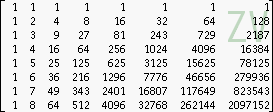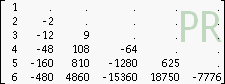# Gottfried Helmshttps://go.helms-net.de D - Kassel  Dez 2006

# Numbertheoretical matricesIn this page I'm presenting a collection of basic numbertheoretical matrices, for instance the Pascalmatrix (binomialmatrix), matrices of Bernoulli-/Euler- and other polynomials, Stirling-numbers and some interesting and systematic relations between them. (The matrices are lower-triangular number-arrays of infinite dimension.)

This collection is primarily a compilation of that matrices and their basic properties. Then their relations are presented and discussed. The occuring identities are in most cases well known, but especially the eigensystem-decompositions (and their consequences) seem to be unique in this collection.

Most of the relations were found heuristically by playing around with that matrices in the style of experimenting with a toolbox. The proofs for the given identities and relations shall be added as I have time (and as far as I'm able to derive them). When I was looking in literature (mostly internet resources) I found most of these identities spreaded over various resources: here I try to put them together in a more systematic context.

An unexpected but interesting extension popped up very soon: the problems of divergent summation: matrix-operations with that (in most cases) integer-numberarrays means to assign values to divergent summation in a very natural way.

Also the references section is far from being complete. I'll add the references while I'm extending the already present chapters as well while I'm creating the missing ones.

Gottfried Helms 12'2006 - 06'2008## Intro and notation

The basic definitions and conventions, the short names of the basic matrices.

Intro

## 1 Basic matrices and properties

The signed Pascal-matrix and their eigensystems (not yet ready)

A generalization of the subdiagonal/matrix-exponential-approach leads to versions of the Laguerre-matrix and other, yet anonymous matrices.

The fundamental matrices of Bernoulli/Euler-polynomials BY/EY, the (Jacob Bernoullian) matrices G (Gp & Gm) and the (Eulerian) matrix Ep

Stirlingnumbers : the matrices St1 and St2 of Stirlingnumbers of 1st and 2nd kind, Bell-numbers. (11.02.07)## 2 More matrix-relations of interest

The Vandermondematrix ZV seems more and more the core matrix of all that considerations. This is not surprising: it is vertically read the matrix of zeta-sequences and horizontally read of the geometric series: the most basic aspects that may be studied in number-theories.## 3 Special matrices and interesting details

The "Gaussian"-matrix occured to me when I tried to find derivatives and integrals of the gaussian function. The matrix is then the triangle of coefficients of z which are needed to express the derivatives. It occured, that even this matrix is closely related to the binomialmatrix by the same subdiagonal/matrix-exponential-scheme as a very nice and simple generalization.

Generalized Bernoulli-Recursion: a surprising recursion formula to define the Bernoulli-numbers. This formula can be parametrized, and very natural selections of parameters lead to a whole family of numbertheoretical sequences, which shows a basic simple relationship between harmonic, geometric, constant, reciprocal binomial series together with the bernoulli-numbers and "eta"-numbers. (14.02.07)

Zeta-sums: a couple of sums-of-bernoulli-numbers, sums-of-zeta-values- identities

Sums of like powers: an approach to find an equivalent to bernoulli-polynomials by means of matrix-algebra. The Faulhaber/Bernoulli-matrix is recovered by elementary means and the zeta-connection re-established (20.5.2007).## 4 divergent Summation using matrices

Summation:Introductory remarks about divergent summation: Cesaro/Euler-summation in matrix-representation; A direct summation-approach for some special applications

Bell-/Stirling numbers and (divergent) alternating sums of their sums (1.6.2008)

A formula for infinite "geometric series" of Power-towers is presented.

The formula seems to allow to extend the domain, as it is known for infinite powertowers, to values s>e1/e (4.7.2007)## Older versions:

Pascalmatrix : introduces the concept, the Pascalmatrix, Gp-matrix as eigensystem, St2/St1-matrices; can be used until the chapter "Bernoulli-/GP-matrix" is rewritten. (German version)

Summation: divergent summation using the binomial and other matrices / still manuscript version. Touches the most aspects which are discussed in the newer articles in terms of heuristic findings and propositions.